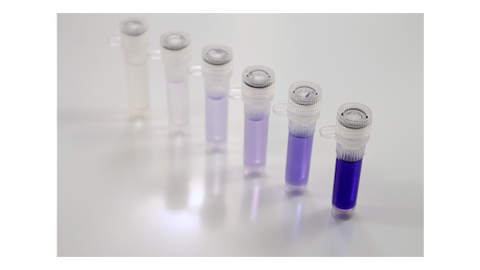labservices@ionizationlabs.com | 737-231-0772 | TDA #2020003

# Understanding Dilutions

In this article, we explain the importance of dilution as it applies to analytical chemistry, what dilution factors are, and specific equations and calculations to use for concentration and volume determination.###### Figure 1. A picture depicting a serial dilution

Dilution is the process of decreasing the concentration of solutes in a solution by adding or mixing in solvent. During dilution, solvent such as water, methanol, isopropyl alcohol is introduced to a solution which ultimately decreases the concentration of the solution. In analytical chemistry, solutes may be the sample that is being prepared or the analyte of interest. In general, solute is the minor component inside a solution. It is the material that gets dissolved into the solvent to create the solution.###### Figure 2. An image showing the difference between solute, solvent, and solution.

Dilutions are oftentimes a required step during the sample preparation process to ensure the analytes are prepared at a concentration that matches the calibration range of the analytical instrument that is being utilized.

There are several equations that are relevant towards dilutions. Below you will find equations and examples that are specific to dilution.

Dilution Equation

C1*V1=C2*V2
C1 = Initial Concentration
V1= Initial volume
C2 = Final Concentration
V2 = Final Volume

The dilution equation allows us to calculate how to dilute solutions to various concentrations or volumes.

Example: You start off with 1 mL ampoule of a 1mg/mL solution and are trying to formulate a calibration level at 25 μg/mL. How much volume can you make if you use the entire 1mL ampoule.

C1*V1=C2*V2 where:
C1= 1mg/mL = 1000μg/mL
V1= 1mL
C2= 25μg/mL
V2= ?
(C1*V1)/C2=V2
(1mL*1000ug/mL)/(25ug/mL)=V2
40mL=V2
Dilution Factor Equation
Dilution Factor = Final Volume/Solute Volume

Dilution factor or dilution ratio is used to describe how much of the original stock solution is present in the total solution after dilution. In other words, it provides the ratio of the initial, undiluted volume of solution to the final diluted solution. Below are some examples to determine dilution factors. Dilution factor is the reciprocal or inverse of dilution ratio.

Overall Dilution Factor

For multiple dilutions, the dilution factor is the product of the dilution factors for each individual dilution.

DFoverall=DF1*DF2*DFn
DFoverall =  The overall dilution factor for the process
DF1 = The first dilution factor in the process
DF2 = The second dilution factor in the process
DFn = The nth dilution factor in the process

Example: Add 100μL of a tincture sample to 1 mL of extraction solvent. What is the dilution factor?

1mL = 1000μL
Solute volume = 100μL of sample
Final volume = sample (100μL) and solvent (1000μL) = 1100μL
Dilution Factor = (1100μL)/(100μL)= 11
The process has a dilution factor of 11.

Example: 100mg of 100% reagent grade anhydrous caffeine is dissolved in 10mL of methanol. Next, 150μL of the dissolved caffeine solution is transferred to an HPLC vial containing 1.5mL of methanol. What is the total dilution for the caffeine inside the HPLC vial?

In the first step, 100mg of caffeine is dissolved in 10 mL of methanol. This results in the caffeine being diluted 10X its original concentration. Secondly, 150 μL gets transferred into 1500μL for a dilution of 11X. To get the total dilution or the overall dilution factor for the caffeine, you would multiply the first dilution factor by the second dilution factor to get 110X. Thus, the caffeine was diluted 110X its original concentration.

Useful Definitions Pertaining to Dilution:

Sample - A representative piece of material that is used and prepared for analytical analysis

Solute - A substance that is dissolved in solvent. It is the minor component in a solution. During sample preparation, analytes that are extracted can be considered the solutes in the solution.

Solvent - A liquid that is used to dissolve the solute. Some common examples of solvents include ethanol, acetone, methanol, acetonitrile, isopropyl alcohol, and water.

Solution - A liquid mixture that contains the minor component, solute, and major component, solvent.

Analyte - A substance or chemical material that is being identified or quantified. Analytes are molecules that are analyzed by laboratories.

Dilution - The process of decreasing the concentration of a solute in a solution. This is done by adding more solvent to the solution.

Diluent - A substance used to dilute something. While close to a solvent, diluents are liquids used In some cases, diluent can also be referred to as solvent.

Dilution Factor - The total volume of solution per aliquot volume.

Resources:

https://toptipbio.com/c1v1-equation/

https://www.csus.edu/indiv/t/toofanj/jthome/students/chem-31/CH31-Subjects/Dilution-FactorXX.pdf